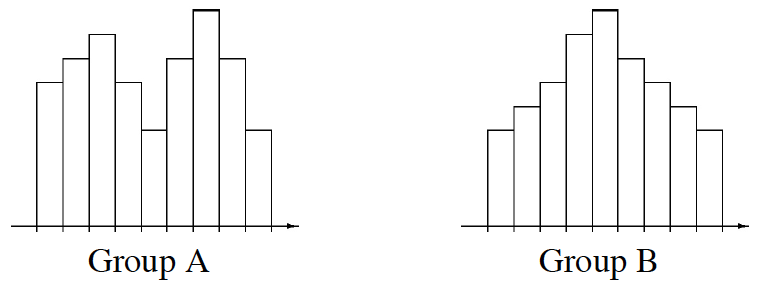Home > CC2MN > Chapter cc18 > Lesson cc18.1.3 > Problem8-39

8-39.

Assume that the histograms below represent the amount of time it took two different groups of $100$ people to run a $5$K race. Assume that the mean of each histogram is the same. Which group has a greater mean absolute deviation? Why? Homework Help ✎Group B has more data points near the center. Group A has more data points farther from the center.